# Unrealized Gain and Loss

Excel
For the analysis of the investment portfolio, it is helpful to see the unrealized gain or loss. These amounts show how many you will receive or lose if you realize all available stocks right now. Sure, there are some fees for the operations that can decrease gain or increase loss, but, at least, you want to see the least approximate amounts: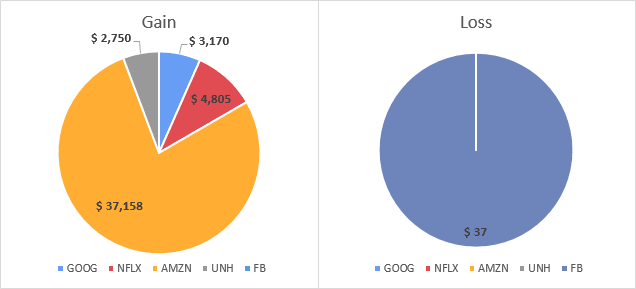To create pie charts like ones above, do the following:

1.   Create or add a data range:

a)   with stock ticker symbols and prices (see How to view current stocks prices and other quotes in Excel).

For example: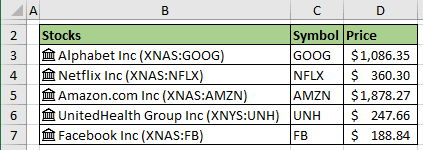b)   with transactions (if you have a fixed quantity, you can add them without an additional calculation).

For example: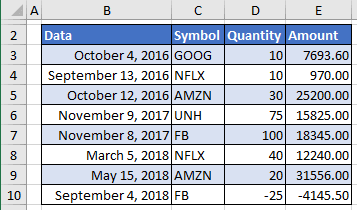2.   Add new columns to calculate current value, Gain and Loss:

• Quantity (if you have fixed quantity, you can add them without additional calculation) using the following formula:

= SUMIFS (Transactions[Quantity], Transactions[Symbol], "="&<symbol>)

where:

• SUMIFS is a function to sum cells that meet multiple criteria:

SUMIFS (<the range to be summed>, <the first range to evaluate>, <the criteria for the first range>, [<the second range>], [<the criteria for the second range>], ...)

• Transactions is a table from ppoint 1.b),
• <symbol> is a stock symbol from column C.

For the cell E4 this formula is:

= SUMIFS (Transactions[Quantity], Transactions[Data], "="&C3):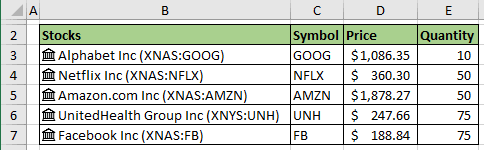• Value using the formula:

= <price> * <quantity>

For the cell F4 this formula is:

= D4 * E4: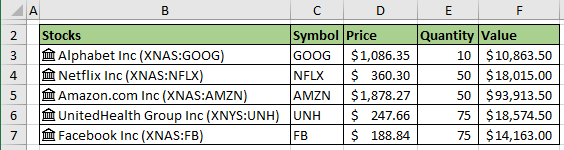Note: You can hide the price and quantity data and use the formula:

= <stock>.Price * SUMIFS (Transactions[Quantity], Transactions[Symbol], "="&<symbol>)

where, <stock>.Price is a reference to the Price field of the Stocks data type.

For example: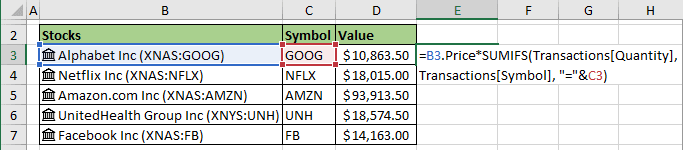• Gain:

= IF ((<value> - <cost>) > 0, <value> - <cost>, 0)

where:

• <value> is a calculated amount from the previous step,
• <cost> can be calculated like a <quantity> using the formula:

= SUMIFS (Transactions[Amount], Transactions[Symbol], "="&<symbol>)

For this example: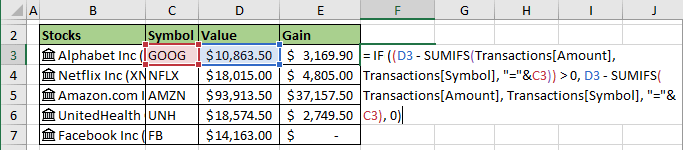• Loss:

= IF ((<cost> - <value>) > 0, <cost> - <value>, 0)

For this example: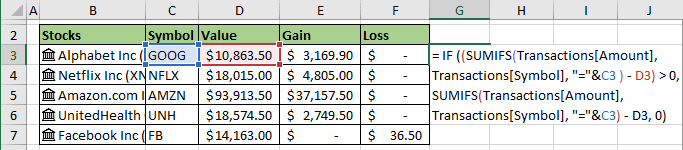3.   Select the data range to create a pie chart.

For this example, C3:C7 and E3:E7.

4.   On the Insert tab, in the Charts group, click the Insert Pie or Doughnut Chart button:Choose the Pie chart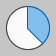: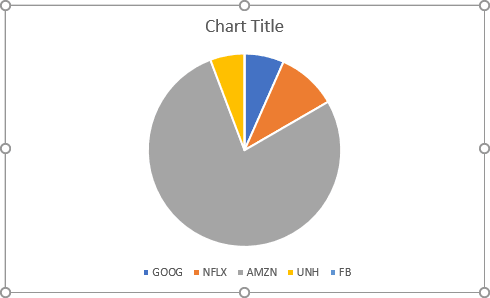Make any other adjustments to get the look you need.